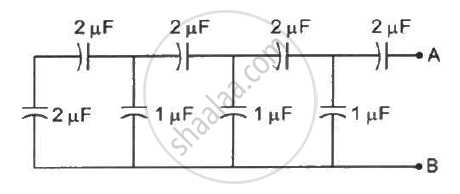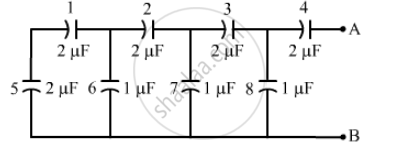Department of Pre-University Education, Karnataka course PUC Karnataka Science Class 12
Share

# Find the Capacitance of the Combination Shown in Figure Between a and B. - Physics

ConceptCapacitors and Capacitance

#### Question

Find the capacitance of the combination shown in figure between A and B.#### SolutionCapacitors 5 and 1 are in series.

Their equivalent capacitance, C_(eq) = (C_1C_5)/(C_1+C_5) =(2 xx 2)/(2+2) = 1  "uF"

therefore C_(eq) = 1

Now, this capacitor system is parallel to capacitor 6. Thus, the equivalent capacitance becomes 1 + 1 = 2 μF

The above capacitor system is in series with capacitor 2. Thus, the equivalent capacitance become (2 xx 2)/(2+2) = 1  "uF"

The above capacitor system is in parallel with capacitor 7. Thus, the equivalent capacitance becomes 1 + 1 = 2 μF

The above capacitor system is in series with capacitor 3. Thus, the equivalent capacitance becomes  (2 xx 2)/(2+2) = 1  "uF"

The above capacitor system is in parallel with capacitor 8. Thus, the equivalent capacitance becomes

1 + 1 = 2 μF

The above capacitor system is in series with capacitor 4. Thus, the equivalent capacitance becomes (2 xx 2)/(2+2) = 1  "uF"

Hence, the equivalent capacitance between points A and B of the given capacitor system is 1 μF.

Is there an error in this question or solution?

#### Video TutorialsVIEW ALL 

Solution Find the Capacitance of the Combination Shown in Figure Between a and B. Concept: Capacitors and Capacitance.
S